## 二项分布(Binomial Distribution)

\begin{equation}
P{X=x}=C_{n}^{x} p^{x} q^{n-x}, \quad x=0,1,2, \cdots, n
\end{equation}

from scipy.stats import binom
import seaborn as sns
data_binom = binom.rvs(n=10,p=0.5,size=10000)
ax = sns.distplot(data_binom,
kde=False,
color='green',
hist_kws={"linewidth": 15,'alpha':1})
ax.set(xlabel='Binomial Distribution', ylabel='Frequency')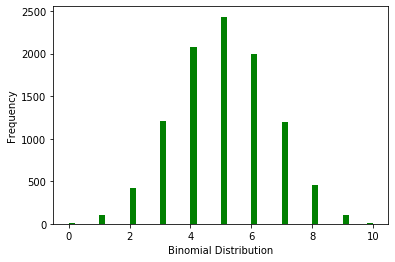# 可以打印随机变量的值，按照定义，其值为出现A事件的次数，范围肯定在[0,1]
print(data_binom)
[2 3 6 ... 5 4 3]

## 贝努里分布(Bernoulli Distribution)

\begin{equation}
\end{equation}

from scipy.stats import bernoulli
data_bern = bernoulli.rvs(size=10000,p=0.5)
ax= sns.distplot(data_bern,
kde=False,
color="green",
hist_kws={"linewidth": 15,'alpha':1})
ax.set(xlabel='Bernoulli Distribution', ylabel='Frequency')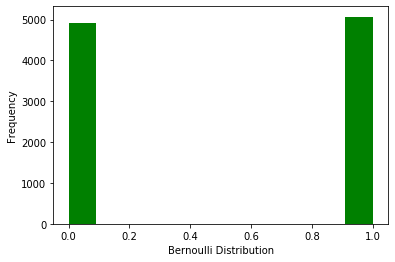## 几何分布(Geometric distribution)

 几何分布的特点：
（1）进行一系列相互独立的试验；
（2）每一次试验既有成功的可能，也有失败的可能，且单次试验的成功概率相同；
（3）主要是为了取得第一次成功需要进行多少次试验。

\begin{equation}
P{X=k}=p (1-p)^{k-1}, \quad k \geqslant 1
\end{equation}

from scipy.stats import geom
data_geom = geom.rvs(size=10000,p=0.5)
ax= sns.distplot(data_geom,
kde=False,
color="green",
hist_kws={"linewidth": 15,'alpha':1})
ax.set(xlabel='Geometric Distribution', ylabel='Frequency')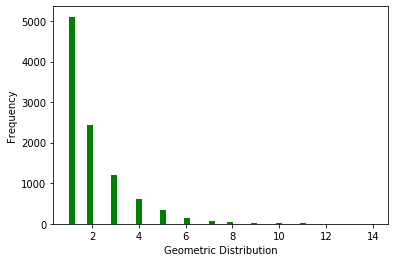## 泊松分布(Poisson distribution)

\begin{equation}
P(X)=\frac{\lambda^{x} \mathrm{e}^{-\lambda}}{x !}, \quad x=0,1,2, \cdots
\end{equation}

from scipy.stats import poisson
data_poisson = poisson.rvs(mu=3, size=10000)
ax = sns.distplot(data_poisson,
bins=30,
kde=False,
color="green",
hist_kws={"linewidth": 15,'alpha':1})
ax.set(xlabel='Poisson Distribution', ylabel='Frequency')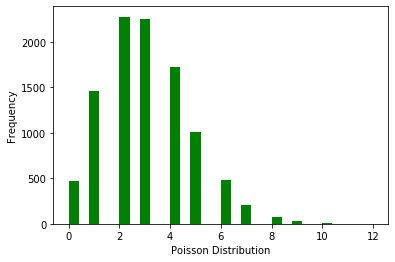## 正态分布(Normal Distribution)

\begin{equation}
f(x)=\frac{1}{\sigma \sqrt{2 \pi}} \mathrm{e}^{-\frac{1}{2 \sigma^{2}}(x-\mu)^{2}}, \quad-\infty<x<+\infty
\end{equation}

from scipy.stats import norm
# 生成标准正态分布，N(0,1)
data_normal = norm.rvs(size=10000,loc=0,scale=1)
ax = sns.distplot(data_normal,
bins=100,
kde=True,
color="green",
hist_kws={"linewidth": 15,'alpha':1})
ax.set(xlabel='Normal Distribution', ylabel='Frequency')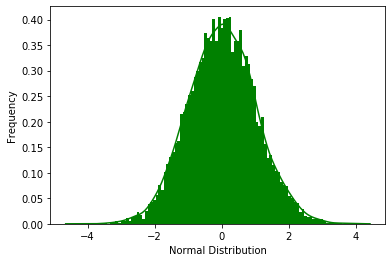## 总结posted on 2019-12-01 21:41  camash  阅读(...)  评论(...编辑  收藏

• 随笔 - 87
• 文章 - 0
• 评论 - 8
• 引用 - 0